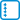## Extension of orthonormal bases

For the following matrix $$\mathbf A$$ find an orthonormal basis of $$\ker (\mathbf A)$$ and then extend it to an orthonormal basis of $$\mathbb R^5$$.

$\mathbf A = \begin{pmatrix} 2 & 0 & 3 & -6 & 0 \\ -5 & 0 & 3 & 8 & 0 \\ 7 & 4 & 0 & -14 & 3 \\ -1 & 1 & -12 & 10 & 7 \\ 5 & 7 & -3 & -8 & -1 \end{pmatrix}$

Also find an orthonormal basis of $${\mathcal R} (\mathbf A)$$ and then extend it to an orthonormal basis of $$\mathbb R^5$$.

How do the found bases relate to each other?

• #### Solution

By Gaussian elimination we calculate that the matrix $$\mathbf A$$ has the rank $$4$$, therefore $$\dim(\ker(\mathbf A))=1$$ and backward substitution we get $$\ker(\mathbf A)=\mathcal L (\{(6{,}0,2{,}3,0)^T\})$$.

After normalization, we get the orthonormal basis of the kernel $$X_1=\left\{\left(\frac{6}{7},0,\frac{2}{7},\frac{3}{7},0\right)^T\right\}$$.

If we extend $$X_1$$ to a basis of $$\mathbb R^5$$ e.g. by the second to fifth vectors of the standard basis and perform Gram-Schmidt orthonormalization, we get the basis extension $$Y_1=\left\{ \mathbf e^2, \left(\frac{-4}{\sqrt{245}},0,\frac{15}{\sqrt{245}},\frac{-2}{\sqrt{245}},0\right)^T, \left(\frac{-1}{\sqrt{5}},0{,}0,\frac{2}{\sqrt{5}},0\right)^T, \mathbf e^5 \right\}$$

To obtain an orthonormal basis of $$\mathcal R (\mathbf A)$$ we apply Gram-Schmidt orthonormalization to the rows of the matrix $$\mathbf A$$, thus obtaining the basis $$Y_2=\left\{ \left(\frac{2}{7} , 0 , \frac{3}{7} , \frac{-6}{7} , 0 \right)^T, \left(\frac{-3}{7} , 0 , \frac{6}{7} , \frac{2}{7} , 0 \right)^T, \left(0 , \frac{4}{5} , 0 , 0 , \frac{3}{5} \right)^T, \left(0 , \frac{-3}{5} , 0 , 0 , \frac{4}{5}\right)^T \right\}$$.

To extend it to an orthonormal basis of $$\mathbb R^5$$ it is enough to take any vector linearly independent of the vectors of $$Y_2$$ e.g. the vector $$\mathbf e^5$$ and To obtain the Gram-Schmidt orthonormalization from it the extension $$X_2=\left\{\left(\frac{6}{7},0,\frac{2}{7},\frac{3}{7},0\right)^T\right\}$$

Since $$\ker (\mathbf A)$$ is the orthogonal complement of $$\mathcal R (\mathbf A)$$, $$Y_2$$ is also a basis of $$\mathcal R (\mathbf A)$$ as well as $$X_2$$ is a basis of $$\ker (\mathbf A)$$ (in our case indeed $$X_1 = X_2$$).

In fact, it would be possible to take the basis $$\mathcal R (\mathbf A)$$ as $$Y_3=\left\{ \left(\frac{2}{7} , 0 , \frac{3}{7} , \frac{-6}{7} , 0 \right)^T, \left(\frac{-3}{7} , 0 , \frac{6}{7} , \frac{2}{7} , 0 \right)^T, \mathbf e^2, \mathbf e^5\right\}$$.